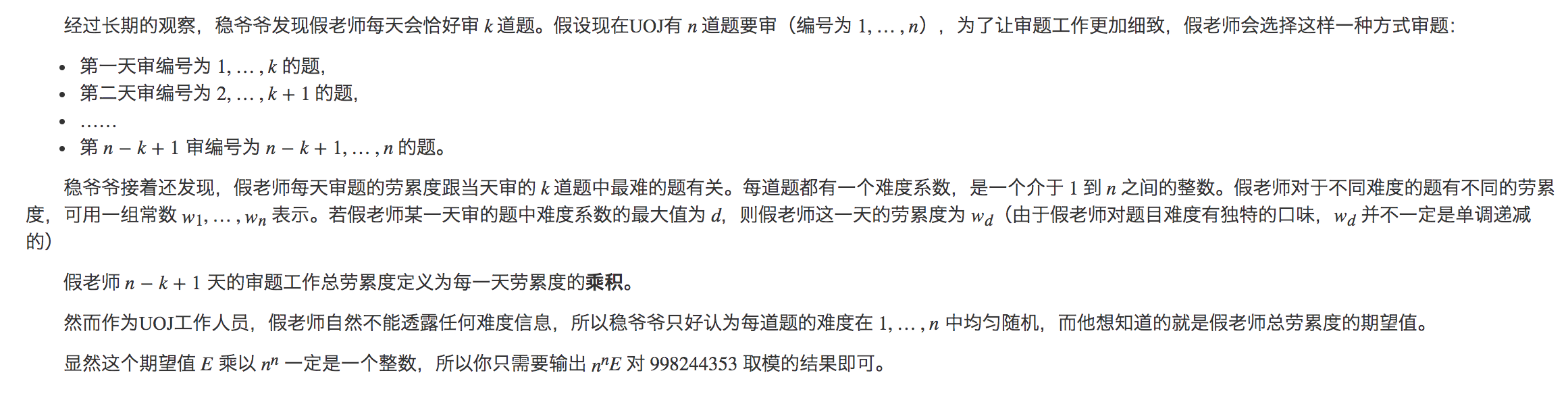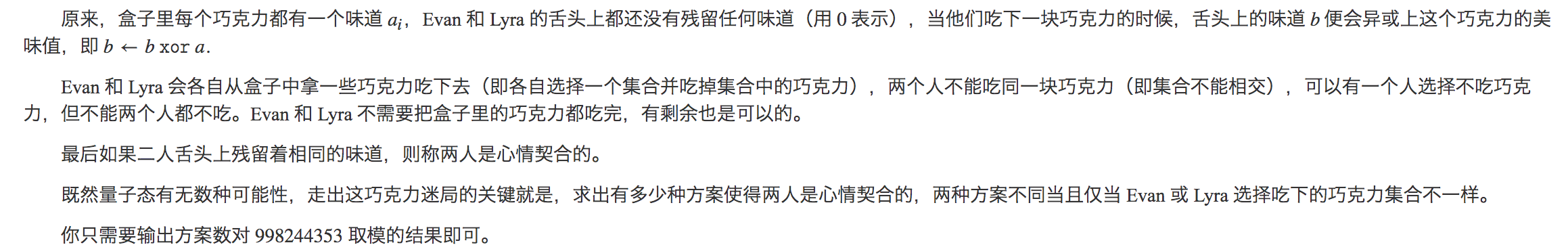## LOJ575 「LibreOJ NOI Round #2」不等关系

f_{i, j} = \begin{cases} \displaystyle \sum_{k=1}^{i-1} f_{i-1, k} & (s_{i - 1} = \textrm{<}) \\ \displaystyle \sum_{k=i}^j f_{i-1, k} & (s_{i - 1} = \textrm{>}) \\ \end{cases}

dp_n = \begin{cases} 1 & (n = 0) \\ \displaystyle \sum_{j=0}^{i-1} [j = 0 \textrm{ or } s_j = \textrm{<}] (-1)^{cnt_{i-1} - cnt_j} \frac {dp_{j}} {(i - j)!} & (n > 0) \\ \end{cases}

## LOJ573 「LibreOJ NOI Round #2」单枪匹马

\left[\begin{matrix} a & 1 \\ 1 & 0 \\ \end{matrix}\right] \left[\begin{matrix} x \\ y \\ \end{matrix}\right] = \left[\begin{matrix} ax + y \\ x \end{matrix}\right]

\left[\begin{matrix} 0 & 1 \\ 1 & -a \\ \end{matrix}\right] \left[\begin{matrix} ax + y \\ x \\ \end{matrix}\right] = \left[\begin{matrix} x \\ y \\ \end{matrix}\right]

\begin{aligned} Ans_{l, r} &= \left[\begin{matrix} a_l & 1 \\ 1 & 0 \\ \end{matrix}\right] \left[\begin{matrix} a_{l + 1} & 1 \\ 1 & 0 \\ \end{matrix}\right] \cdots \left[\begin{matrix} a_r & 1 \\ 1 & 0 \\ \end{matrix}\right] \left[\begin{matrix} 1 \\ 0 \end{matrix}\right] \\ &= \left[\begin{matrix} 0 & 1 \\ 1 & -a_{l - 1} \\ \end{matrix}\right] \left[\begin{matrix} 0 & 1 \\ 1 & -a_{l - 2} \\ \end{matrix}\right] \cdots \left[\begin{matrix} 0 & 1 \\ 1 & -a_1 \\ \end{matrix}\right] \left[\begin{matrix} a_1 & 1 \\ 1 & 0 \\ \end{matrix}\right] \left[\begin{matrix} a_2 & 1 \\ 1 & 0 \\ \end{matrix}\right] \cdots \left[\begin{matrix} a_r & 1 \\ 1 & 0 \\ \end{matrix}\right] \left[\begin{matrix} 1 \\ 0 \end{matrix}\right] \end{aligned}

\begin{aligned} F_n &= \left[\begin{matrix} a_1 & 1 \\ 1 & 0 \\ \end{matrix}\right] \left[\begin{matrix} a_2 & 1 \\ 1 & 0 \\ \end{matrix}\right] \cdots \left[\begin{matrix} a_n & 1 \\ 1 & 0 \\ \end{matrix}\right] \\ G_n &= \left[\begin{matrix} 0 & 1 \\ 1 & -a_{n} \\ \end{matrix}\right] \left[\begin{matrix} 0 & 1 \\ 1 & -a_{n - 1} \\ \end{matrix}\right] \cdots \left[\begin{matrix} 0 & 1 \\ 1 & -a_1 \\ \end{matrix}\right] \end{aligned}

## 洛谷4643 [国家集训队]阿狸和桃子的游戏

• 如果两边都是黑点，对答案的贡献为 1 ，可以看做两个黑点分别为答案贡献了 \frac 1 2
• 如果两边都是白点，对答案的贡献为 -1 ，可以看做两个白点分别为答案贡献了 - \frac 1 2
• 如果一边是白点一边是黑点，贡献为 0 ，可以看做黑点为答案贡献了 \frac 1 2 的边权，白点为答案贡献了 - \frac 1 2 的贡献

## LOJ6674 赛道修建

\clubsuit 是 OI 国马拉松协会的会长。有一天，他打算举办全国青少年马拉松奥林匹克联赛（National Olympiad in Marathon in Provinces, NOMP），于是准备在 OI 国中修建一条赛道。

OI 国有 n (n \leq 5 \times 10^4) 个城市，一共有 n - 1 条道路将它们连通。也就是说，OI 国的结构是一棵树。

\clubsuit 会选择一个城市作为起点，再选择一个城市作为终点，这样赛道的长度就等于起点到终点路径上的边数。由于 OI 国的群众非常懒，所以赛道长为 0 也是允许的。

## CTT2018 esperar（PKUSC2019 试机题）

n \leq 100,\ a_i \leq 10^9

## 洛谷5437 【XR-2】约定

ans = \frac{2 n^{n-3}}{n^{n-2}} \sum_{i=1}^{n} \sum_{j=i+1}^{n} (i+j)^k = \frac 2 n \sum_{i=1}^n \sum_{j=i+1}^n (i+j)^k

\begin{aligned} \sum_{i=1}^n \sum_{j=i+1}^n (i+j)^k &= \frac 1 2 \left( \sum_{i=1}^n \sum_{j=1}^n (i+j)^k - \sum_{i=1}^n (i+i)^k \right) \\ &= 2^{-1} \sum_{i=1}^n \sum_{j=1}^n (i+j)^k - 2^{k-1} \sum_{i=1}^n i^k \\ &= 2^{-1} \sum_{i=1}^n \sum_{j=1}^n (i+j)^k - 2^{k-1} f(n, k) \end{aligned}

\begin{aligned} \sum_{i=1}^n \sum_{j=1}^n (i+j)^k &= \sum_{d=1}^{2n} d^k \sum_{i=1}^n \sum_{j=1}^n [i+j=d] \\ &= \sum_{d=1}^n d^k (d-1) + \sum_{d=n}^{2n} d^k \left(2n-(d-1)\right) \\ &= \sum_{d=1}^{n-1} d^{k+1} + \sum_{d=n}^{2n} 2n d^k - \sum_{d=n-1}^{2n-1} d^{k+1} \\ &= f(n-1, k+1) + 2n \left( f(2n, k) - f(n-1, k) \right) - \left( f(2n-1, k+1) - f(n-2, k+1)\right)\\ \end{aligned}

## UOJ311 【UNR #2】积劳成疾## CF487E Tourists